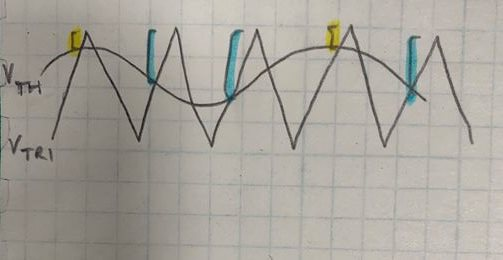If you have a related question, please click the "Ask a related question" button in the top right corner. The newly created question will be automatically linked to this question.

# TLV3502-Q1: tlv3502 overdrive

Part Number: TLV3502-Q1
Other Parts Discussed in Thread: TLV3502

Hi

I am trying to build a PWM generator using your comparator TLV3502 , with analog signal on one side and triangle generator feeding the other input

There is something I don't understand .

The analog voltage and triangle voltage will cross at the same voltage ( + hysterisis  ). The problem is that  propagation speed at 1.2mV overdrive is very small. How can I increase the overdrive ?

• Hi,

I'm not sure if I completely understand your question. To clarify your PWM application:

You are feeding analog signal to non-inverting input, and triangle wave into inverting input. When triangle wave is > Analog signal --> OUTPUT = LOW. When triangle wave is < Analog Signal --> OUTPUT = HIGH.

Are you looking for a way to increase the overdrive of your triangle wave signal in order to have faster propagation delay times? If you have a schematic to share it would be very helpful.

Thanks,

Jonny

• Yes I am asking how to increase the overdrive of my triangle wave

• How are you generating your triangle wave? If using an amplifier to create the triangle wave, I would suggest adjusting the dc bias of the output to increase the overdrive of the signal going into the comparator.

Regards,

Jonny

• Even if I increase the DC bias, voltage of the triangle wave will only trip the comparator when it will be higher than analog input. I fail to understand how this will increase the overdrive

• Hi,

Sorry for the misunderstanding. We define overdrive as the amount of voltage your input signal exceeds your threshold voltage by. Take the example shown below where Vth is your analog signal and Vtri is your triangle wave generator.The highlighted spaces in yellow mark the periods of the triangle wave where the overdrive is very small. Lets assume in this case this overdrive is 1.2mV. However other sections (highlighted in blue) of the triangle wave have a much larger overdrive (>40mV). So if you were to DC bias your triangle wave higher, you would move out of this low overdrive range (Anything less than 20mV the propagation delay becomes highly variable).

By DC biasing your triangle wave, you will get more consistent and accurate PWMing. If I am understanding your case currectly, just biasing your triangle wave by 50mV could get you much more consistent PWMing.

Another suggestion would be to increase the amplitude of your triangle wave to increase the overdrive. Just be sure not to violate the device common mode in this case.

Regards,

Jonny

• Thx you the graph is very clear.

Last question , how many ns the rise should be in the triangle overdrive ? Should it be less than propagation time of the comparator ?

• Thx you the graph is very clear

Last question , how fast should be the rise of the triangle overdrive ? Any relation to propagation time of comparator ?

• You will typically have a faster response if your tr/tf is faster. As long as the tf/tf is symmetric, you should not have a problem with variance in propagation delay.

Just note that with faster rise times you will have a larger duty cycle.

Regards,
Jonny